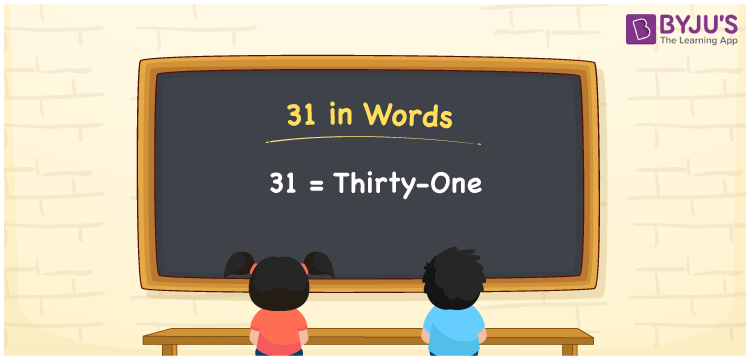# 31 in Words

31 in words can be written as Thirty-one. In this article, you will learn briefly about how to write the number 31 in words. For example, if you buy 5 eggs for Rs. 31 at the provision store, then you can say that “I bought 5 eggs for Thirty-one Rupees at the provision store”. For more ideas about converting numbers in words, make use of the study materials given at BYJU’S. So, the number 31 can be read as “Thirty-one” in words.

 31 in words Thirty-one Thirty-one in Numbers 31

## 31 in English Words## How to Write 31 in Words?

Learn in detail about the place value chart for 31 in words using the table. There are two digits in 31. Given below is the place value chart which shows the place value of the number 31 in a brief way.

 Tens Ones 3 1

The expanded form of 31 is:

3 × Ten + 1 × One

= 3 × 10 + 1 × 1

= 30 + 1

= 31

= Thirty-one

Therefore, 31 in words is written as Thirty-one.

31 is a natural number that precedes 32 and succeeds 30.

31 in words – Thirty-one

Is 31 an odd number? – Yes

Is 31 an even number? – No

Is 31 a perfect square number? – No

Is 31 a perfect cube number? – No

Is 31 a prime number? – Yes

Is 31 a composite number? – No

## Frequently Asked Questions on 31 in Words

Q1

### Write 31 in words.

31 can be written as “Thirty-one” in words.
Q2

### What is the value of 35 minus 4?

The value of 35 minus 4 is 31. Therefore, 31 in words is Thirty-one.
Q3

### Is 31 an even number?

No, 31 is not an even number as it is not completely divisible by 2.